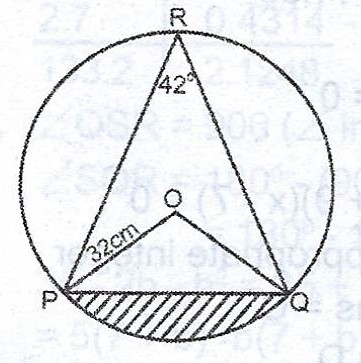# (a) In the diagram, O is the centre of the circle radius 3.2cm. If < PRQ = 42°, calculate, correct to two decimal places, the area of the: (i) minor sector ...

Question 1

(a)In the diagram, O is the centre of the circle radius 3.2cm. If < PRQ = 42°, calculate, correct to two decimal places, the area of the:

(i) minor sector POQ ; (ii) shaded part.

(b) If the sector POQ in (a) is used to form the curved surface of a cone with vertex O, calculate the base radius of the cone, correct to one decimal place.